Physics
Fox

### Summary

• All matter has a property called energy.

• Energy comes in different forms:
•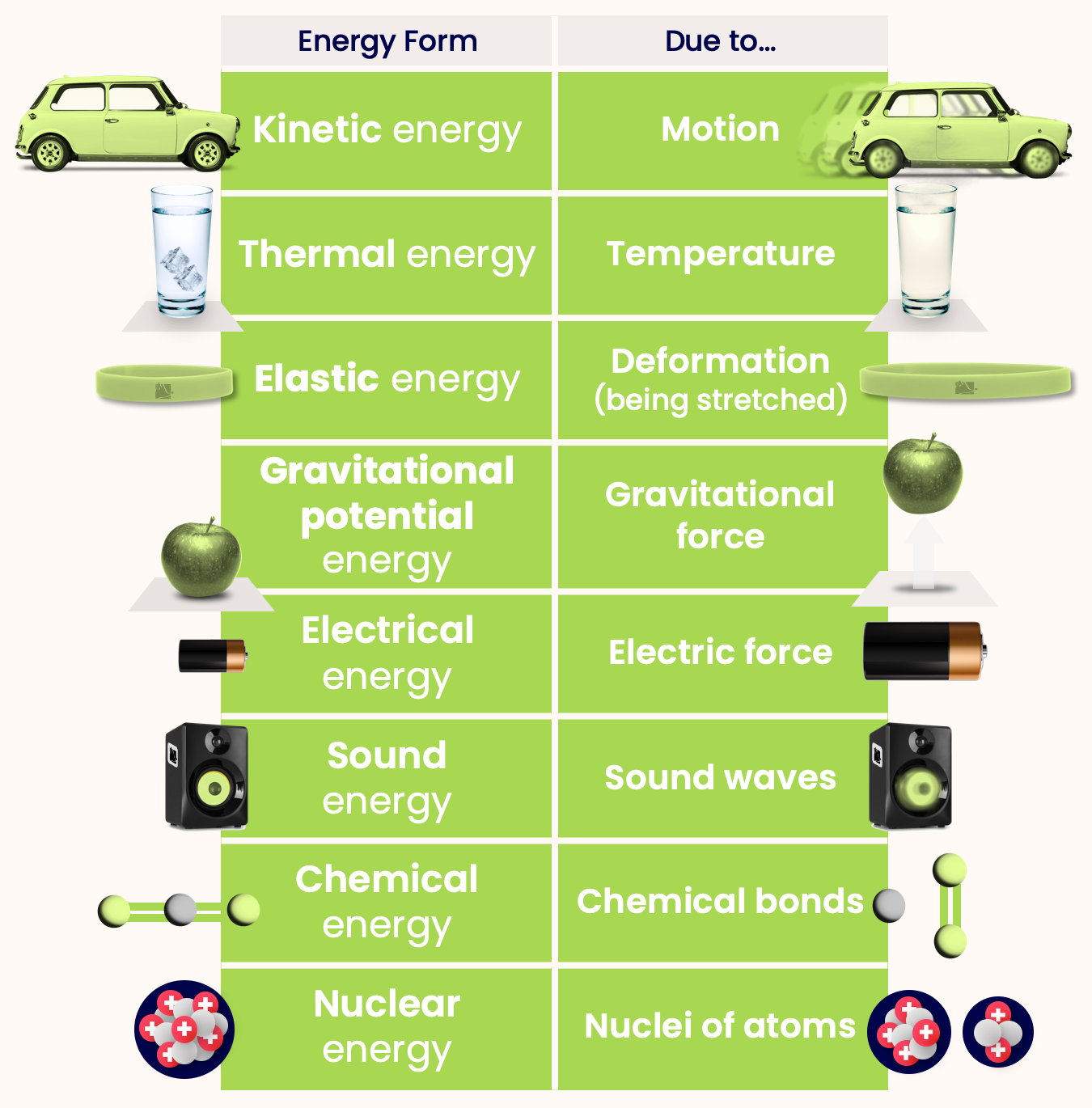• The SI unit of energy is Joules.
•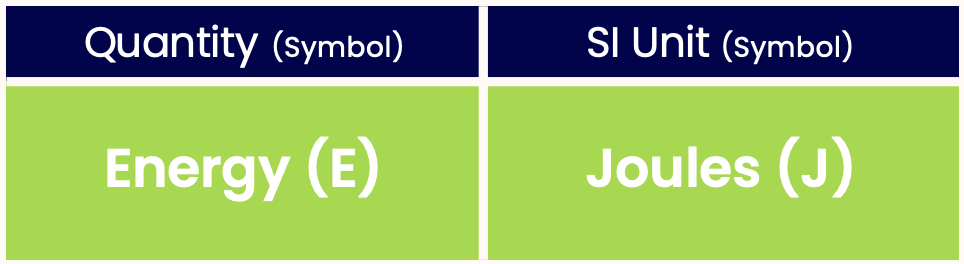I can feel the energy in the air
It feel like I'm supposed to be here

Let's imagine you're in a shop, and there's a box of cereal you want. Tragically, you're too short to reach it. You work up the courage to ask a lanky shopper to help get it for you — how do you describe the box to them?Maybe you'll say what colour it is, how heavy it is, or how tall it is. Maybe even how hot or cold it is (if its near the freezer section).

All these things — colour, weight, height, temperature — are examples of the properties which any object has. Today we're going look at another property of matter, energy.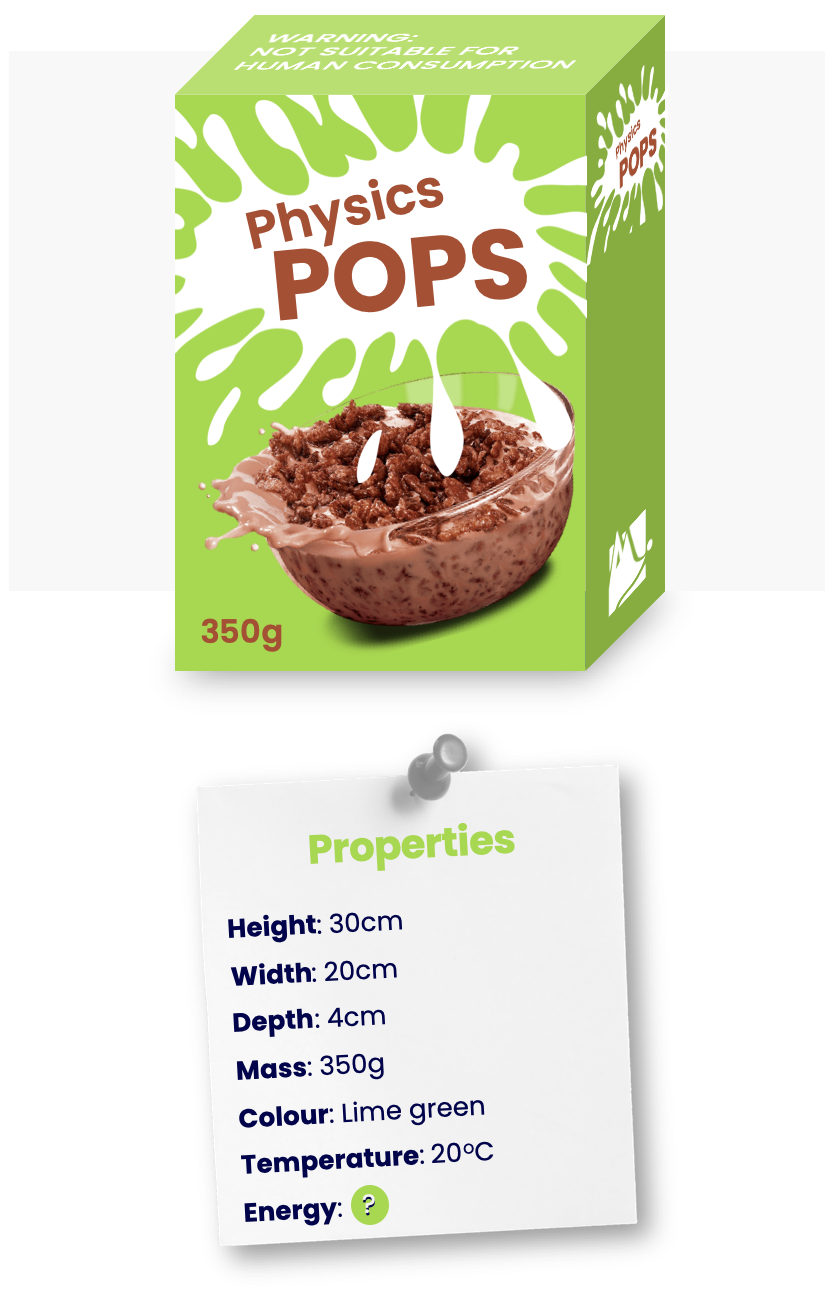Any object has lots of different energies associated with it. These are known as energy forms. Let's have a look at some of them...

Here are some forms of energy that an object might have:

• Kinetic energy — Energy due to the motion of object.
•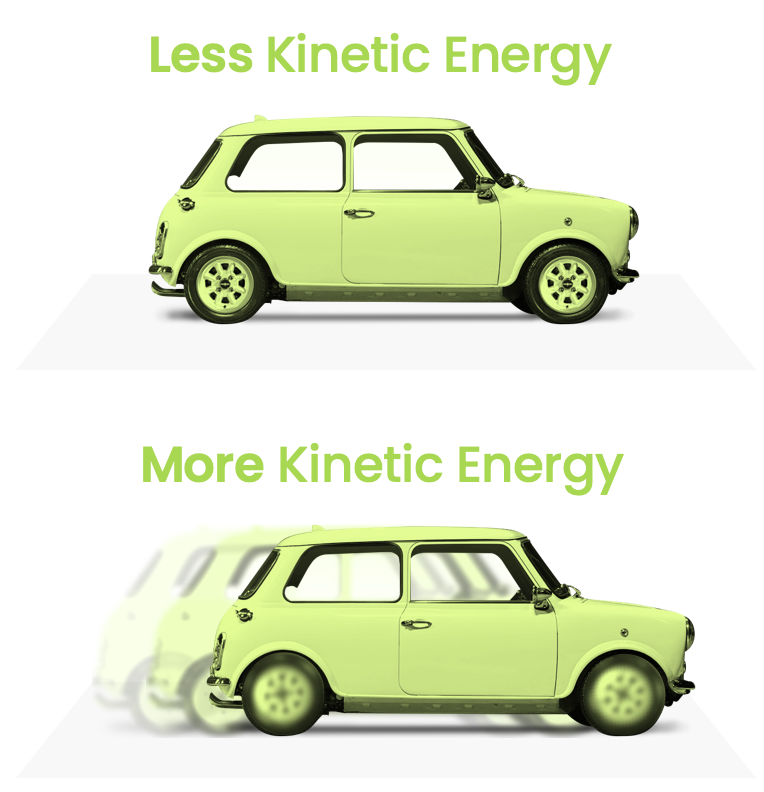• Thermal energy — Energy due to temperature of object
•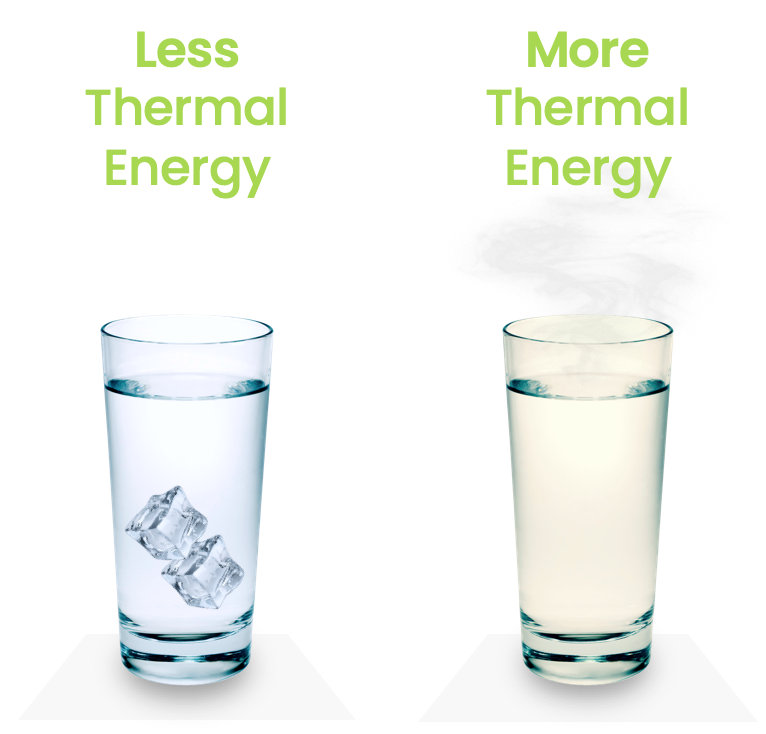• Elastic energy — Energy due to deformation of object.
•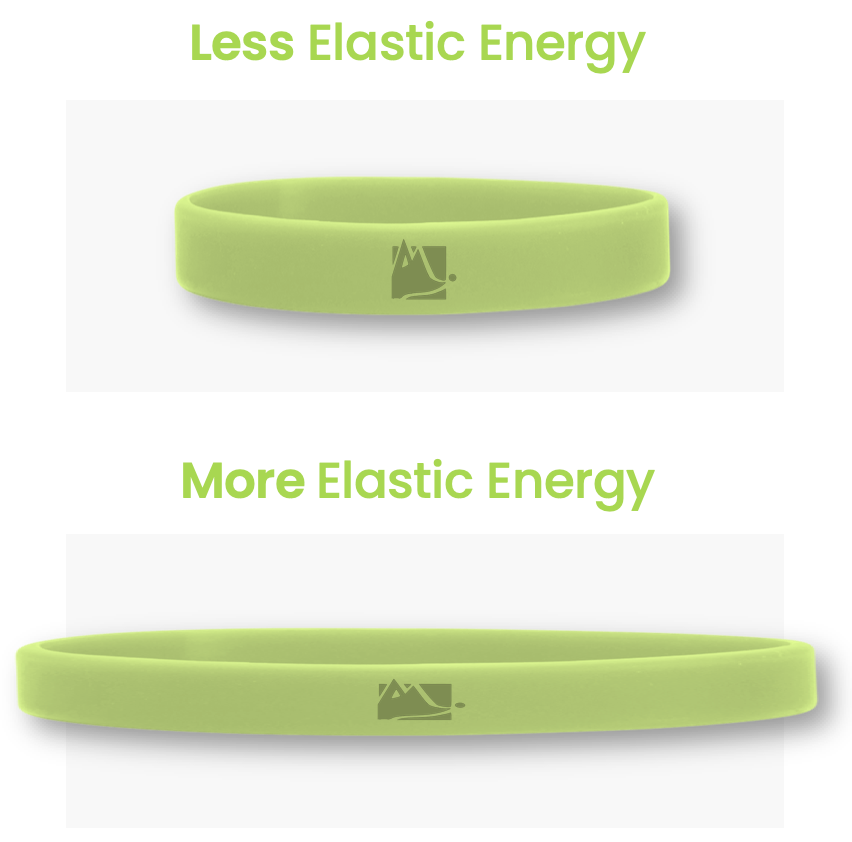• Gravitational potential energy — Energy due to gravitational force on object.
•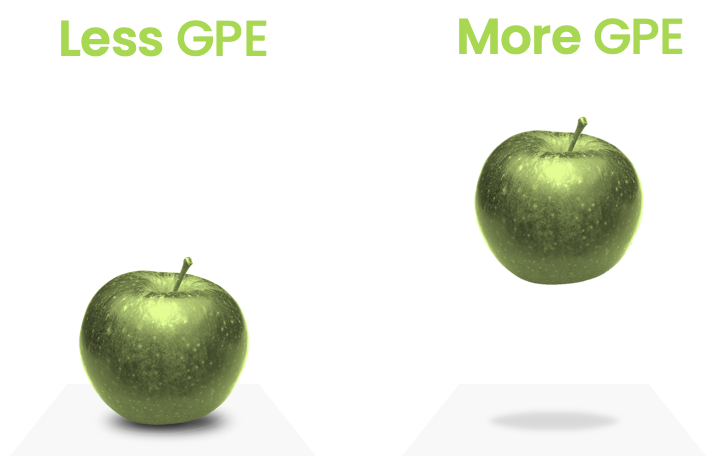• Electrical energy — Energy due to the electric force on an object.
•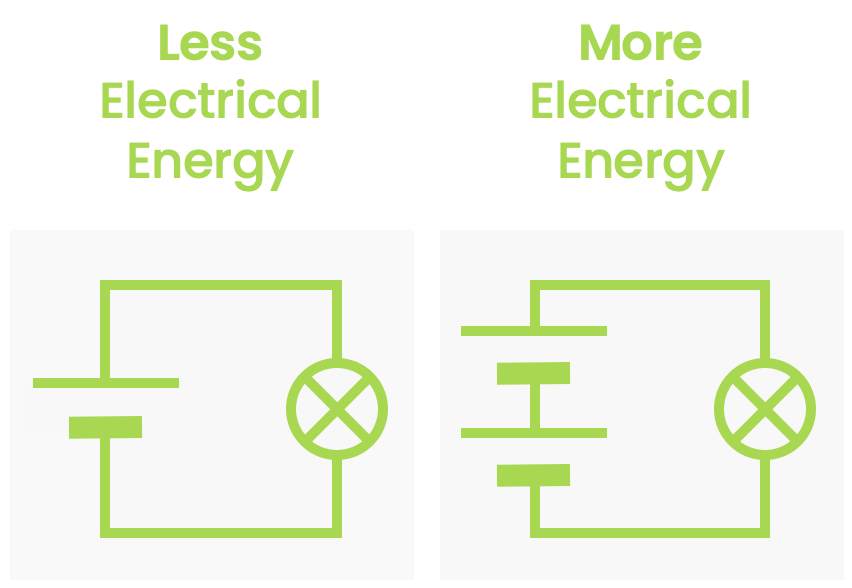• Sound energy — Energy due to sound waves.
•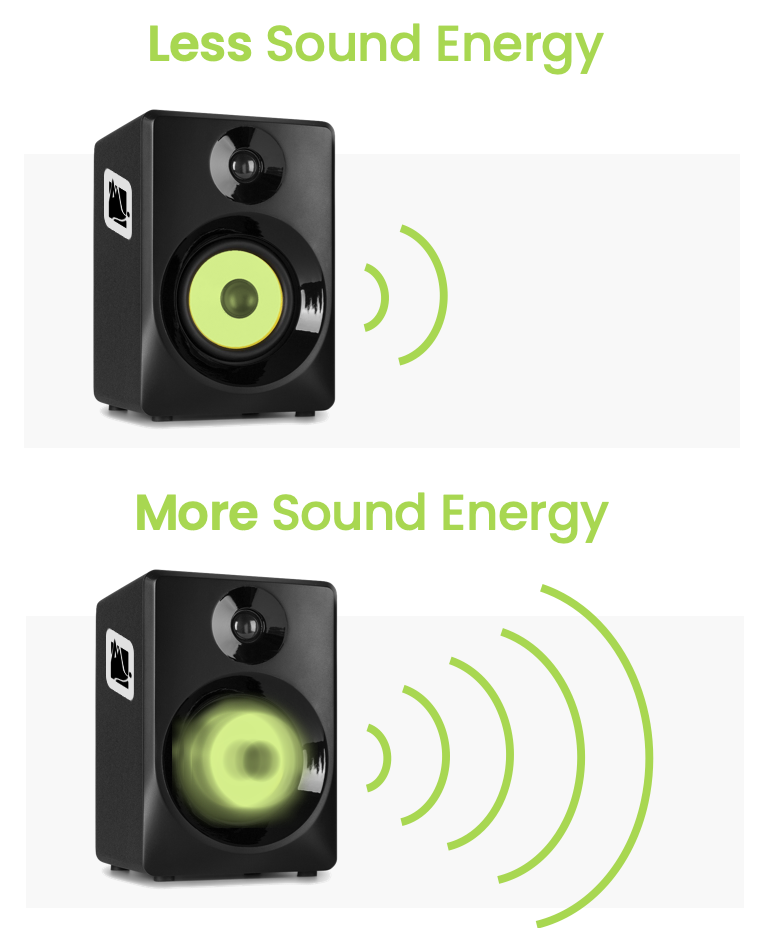• Chemical energy — Energy due to chemical bonds.
•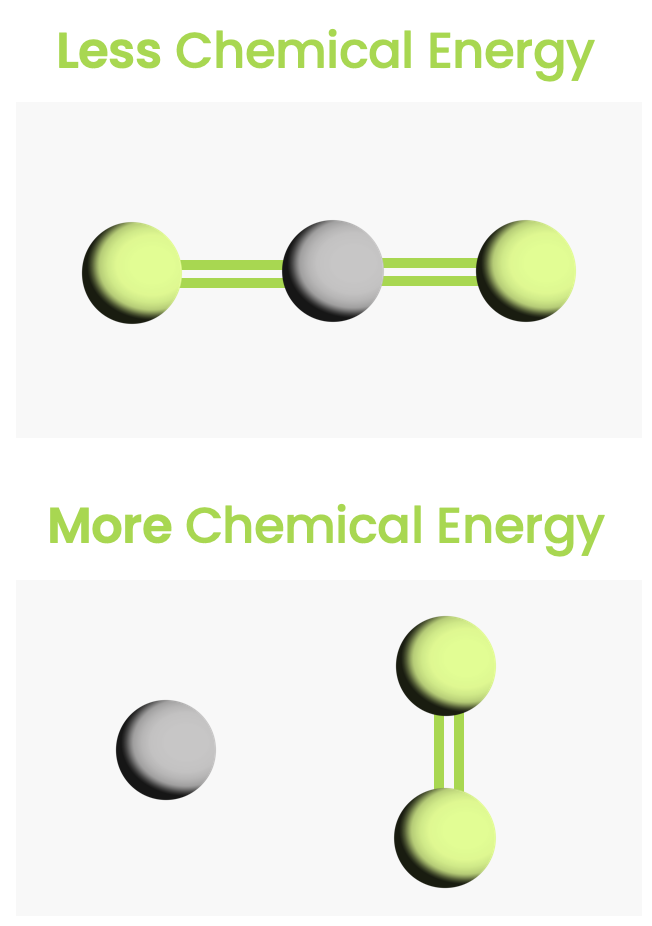• Nuclear energy — Energy in the nuclei of atoms.

(As you can see from all the green links, we will be looking into many of these phenomena in later chapters).

Although the height or temperature of an object is quite easy to find, its energy is not. To find an object's energy we need to do a lot of experiments on it. This makes energy quite an abstract thing. However, just because something is abstract that doesn't mean it's not real or useful.

Like all quantities, energy has a unit. The SI unit of energy is Joules:How much energy is a Joule? Here's how I remember it: it takes roughly 1 Joule to lift an apple by 1 metre.AA batteries each contain about 10,000 J of chemical energy. A car going at 30mph has about 100,000 J of kinetic energy.

Other (non-SI) units of energy include:

• Calorie (used for the energy of food) [1 kcal = 4,200 Joules]
A KitKat is 104 Calories, so it has an energy of 104 × 4,200 J = 437 kJ!

• Kilowatt-hour (used for the energy of electricity in buildings) [1 kWh = 3.6 million Joules]

We now know that all objects have something called energy, which depends on lots of different aspects of the object (such as its speed and temperature).

But we haven't really learnt anything new about nature, have we? Instead I could have said that everything has a ‘wenergy’, and this ‘wenergy’ depends on lots of things. How is this in any way illuminating or useful?

We'll learn why on the next page. As it happens, the most important law of all physics is all about energy.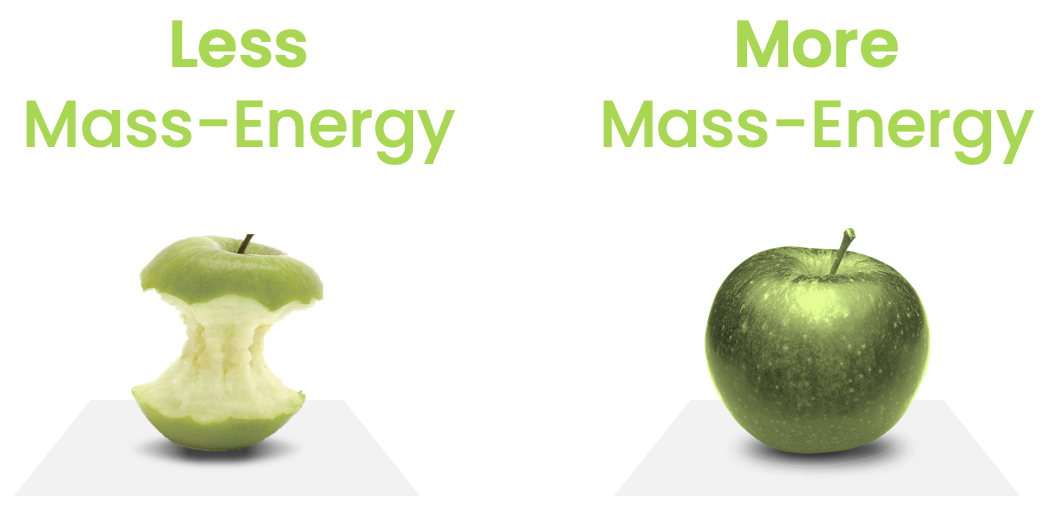There is actually one more type of energy that wasn't listed above. This comes from the mass of an object. For now, we can think of mass as being 'how much stuff' is inside an object — it's what you measure when you weigh yourself on some scales.

The energy of mass is the subject of the world's most famous equation: $E = m c^2$

Where $$m$$ is the mass of an object, and $$c$$ is the speed of light. As we'll learn more about later, $$c$$ in an incredibly large value. So $$c^2$$ is humungous! That means even a tiny amount of mass has an enormous amount of energy.

Try it with your own mass:

kg

So if we could find a way to extract energy from mass, we would have loads of energy! However, it is under quite rare conditions that this mass-energy can be extracted, such as in a star or a nuclear reactor.

Quiz

### Congratulations!

8 of 8 questions completed

+ ⭐️ collected.

• You can change your display name at any time.
• at least 1 letter
• at least 1 number
• at least 8 characters